## 将于1月13日上市 捷途X90内饰官图发布

2019年01月08日 13:52 来源：车行天下 超过：8758次关注

日前，捷途汽车官方发布了旗下全新中型SUV——捷途X90(参数|询价)的官图。此前新车曾在2018北京车展首发，但内饰设计并未正式公开。作为一款中型SUV，捷途X90提供了5/6/7三种座椅布局，此次官图展示的是2+2+2式6座布局版本。另外我们还获悉，新车将于2019年1月13日正式上市。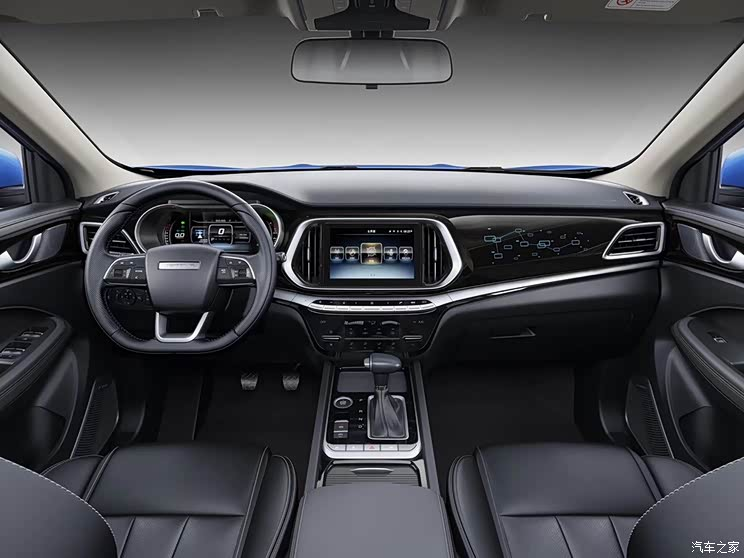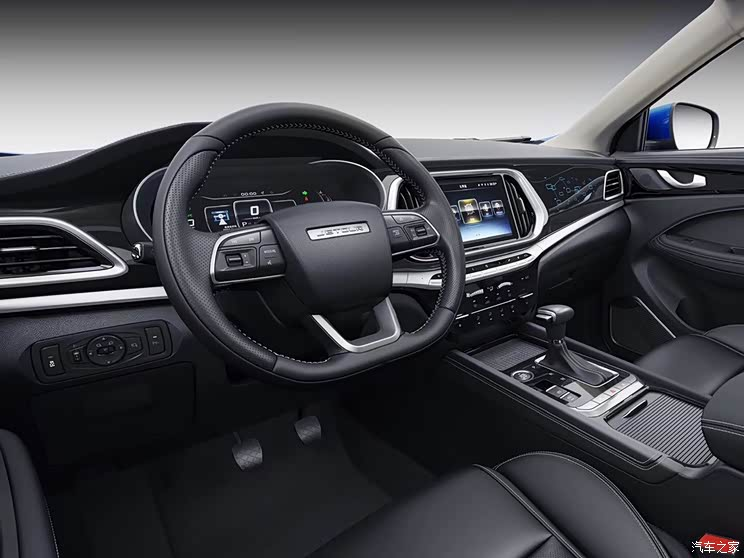从官图来看，捷途X90的内饰采用了纯黑配色，对称式设计的中控台上搭配了恰到好处的哑光银色饰条，辅以简洁的亮黑色饰板和排布整齐的功能控制区域，视觉效果较为时尚。细节方面，新车配备了一块9英寸中控显示屏，同时配备了电子手刹、机械式挡把、一键启动按钮、皮质座椅等。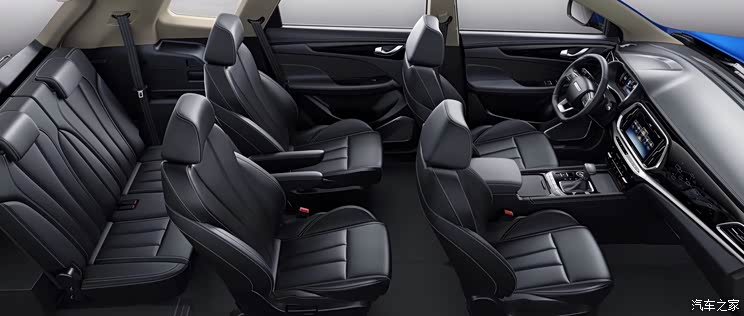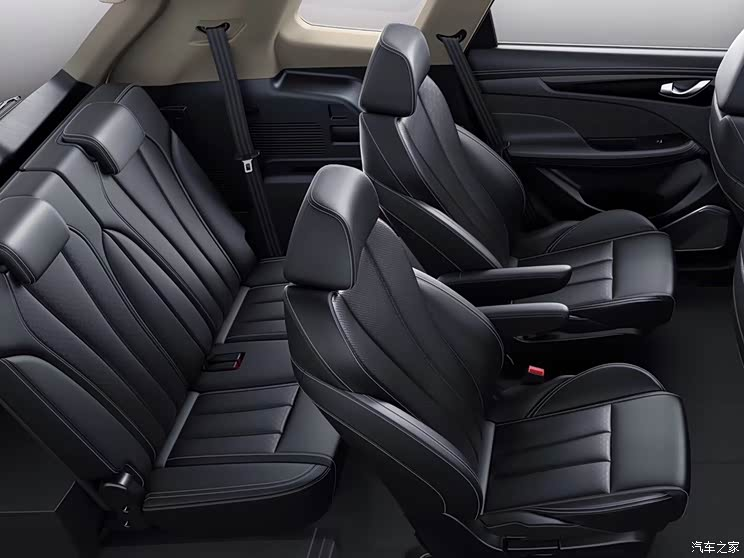根据此前报道，捷途X90将提供5/6/7座三种座椅布局，此次官图中展示的是2+2+2式6座布局。可以看到，该车的第二排采用了两个独立座椅，座椅内侧配备了扶手，中央过道看起来也比较宽敞，便于第三排乘客出入。配置方面参考此前官方信息，新车还提供有后排空调、迎宾踏板/迎宾灯、车道偏离、盲区监测、全景影像、自动雨刷、感应尾门、氛围灯、侧气囊+侧气帘、手机无线充电、4G+Wi-Fi、手环钥匙、自动泊车、指纹钥匙、自适应巡航、流媒体后视镜、自动刹车等多项丰富配置。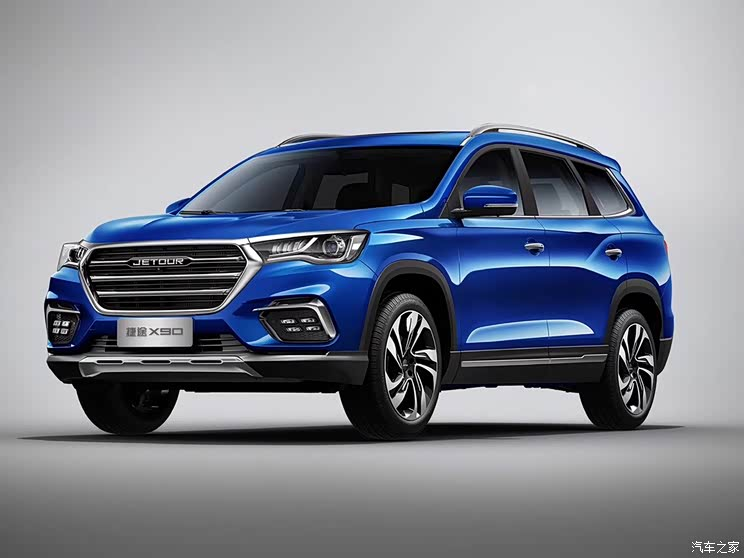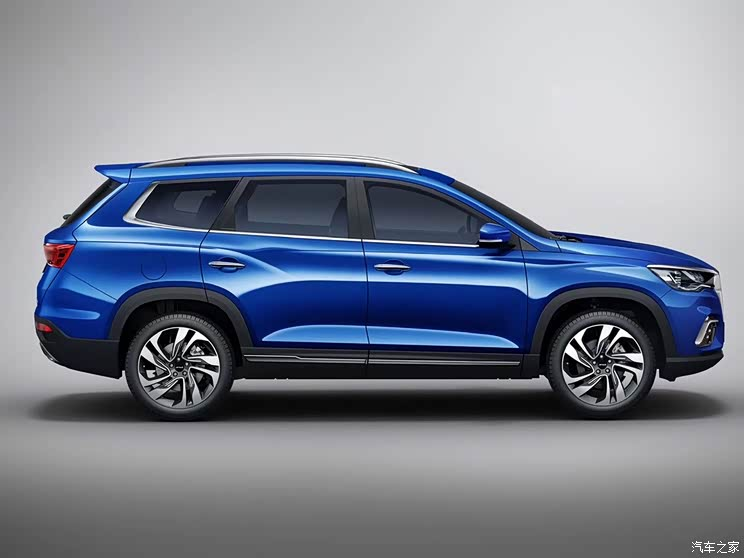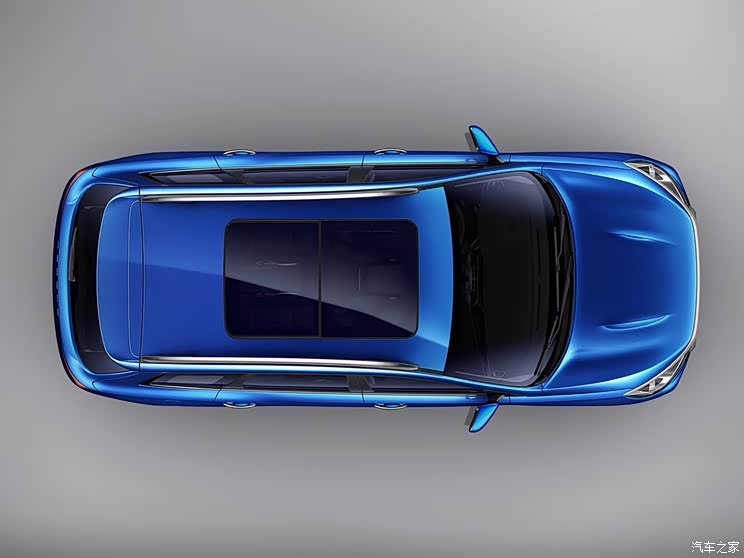回顾外观，捷途X90采用了全新设计语言，前脸盾形进气格栅内部由三根粗壮的横向幅条填充，两侧则与多边形的大灯组相接，营造了大气的整体风格。侧面来看，其车身侧面的线条较为丰富，侧窗边框及黑色包围等处嵌入了镀铬饰条，搭配银色车顶行李架和20英寸双五辐双色轮圈，看上去更显精致。此外，新车还配备了全景天窗。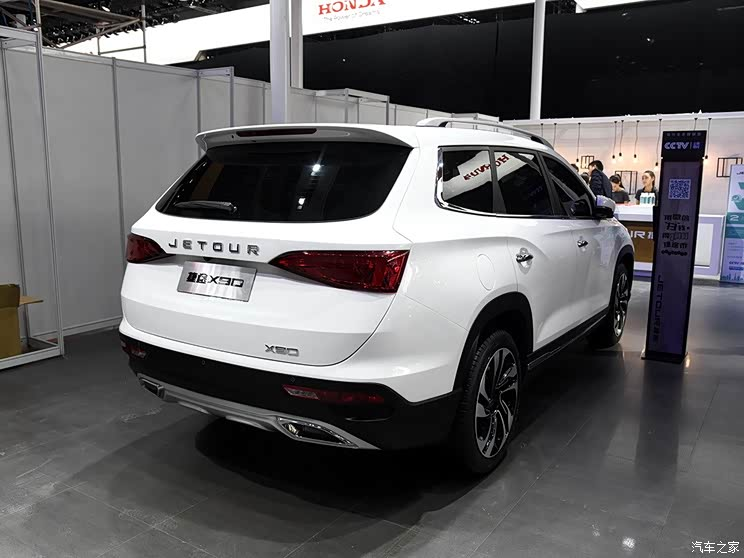车尾方面，新车的造型较为简约，尾窗上方配备有扰流板。与此同时，新车尾门造型立体，横向线条丰富，尾灯造型也较为规整。下方，新车还采用了黑色包围，底部还搭配有银色护板及双边共两出的排气设计。尺寸方面，新车长宽高分别为4840/1925/1745mm，轴距达到2850mm。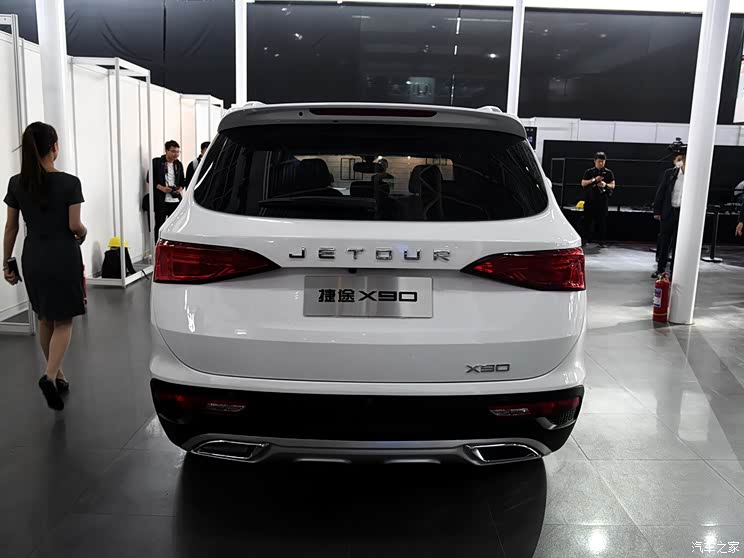动力方面，新车先期仅提供1.5T发动机，最大功率147马力，传动系统预计匹配6速手动或8速手自一体变速箱。后续，新车还将搭载全新的1.6T直喷发动机，传动系统匹配7速双离合变速箱。

#### 相关文章

0-500 字已有评论 0条 查看评论>>

### 热门标签

﻿
• 快速找车
• 选择品牌
• 选择品牌
• A  奥迪
• A  阿斯顿·马丁
• A  阿尔法·罗密欧
• B  宝沃
• B  布加迪
• B  巴博斯
• B  保时捷
• B  宾利
• B  奔驰
• B  宝马
• B  本田
• B  别克
• B  标致
• B  比亚迪
• B  宝骏
• B  北汽制造
• B  北汽新能源
• B  北汽幻速
• B  北汽威旺
• B  北京汽车
• B  奔腾
• B  北汽绅宝
• C  长安
• C  长安商用
• C  长城
• C  昌河
• D  大众
• D  道奇
• D  DS
• D  东南
• D  东风风神
• D  东风风行
• D  东风小康
• D  东风风度
• D  东风
• F  福特
• F  丰田
• F  菲亚特
• F  法拉利
• F  福田
• F  福迪
• F  福汽启腾
• G  观致
• G  广汽传祺
• G  广汽吉奥
• G  GMC
• H  红旗
• H  汉腾汽车
• H  哈弗
• H  哈飞
• H  海格
• H  海马
• H  华颂
• H  黄海
• H  华泰
• H  恒天
• J  吉利汽车
• J  捷豹
• J  Jeep
• J  江淮
• J  江铃
• J  金杯
• J  九龙
• J  金旅
• K  凯翼
• K  凯迪拉克
• K  克莱斯勒
• K  科尼塞克
• K  卡威
• K  开瑞
• L  路虎
• L  林肯
• L  劳斯莱斯
• L  兰博基尼
• L  雷克萨斯
• L  铃木
• L  雷诺
• L  理念
• L  力帆
• L  莲花汽车
• L  猎豹
• L  路特斯
• L  陆风
• M  马自达
• M  MG
• M  MINI
• M  玛莎拉蒂
• M  摩根
• M  迈凯轮
• N  纳智捷
• O  欧宝
• O  讴歌
• O  欧朗
• Q  奇瑞
• Q  起亚
• Q  启辰
• R  日产
• R  荣威
• R  瑞麒
• S  三菱
• S  斯威汽车
• S  萨博
• S  smart
• S  斯柯达
• S  斯巴鲁
• S  思铭
• S  双龙
• S  上汽大通
• S  双环
• T  特斯拉
• T  腾势
• W  沃尔沃
• W  五菱汽车
• W  五十铃
• W  威兹曼
• W  威麟
• X  现代
• X  雪佛兰
• X  雪铁龙
• X  西雅特
• Y  一汽
• Y  英菲尼迪
• Y  英致
• Y  依维柯
• Y  野马汽车
• Y  永源
• Z  众泰
• Z  中华
• Z  中兴
• Z  知豆
• 选择车系
• 选择车系
• 车型对比
• 选择品牌
• 选择品牌
• A  奥迪
• A  阿斯顿·马丁
• A  阿尔法·罗密欧
• B  宝沃
• B  布加迪
• B  巴博斯
• B  保时捷
• B  宾利
• B  奔驰
• B  宝马
• B  本田
• B  别克
• B  标致
• B  比亚迪
• B  宝骏
• B  北汽制造
• B  北汽新能源
• B  北汽幻速
• B  北汽威旺
• B  北京汽车
• B  奔腾
• B  北汽绅宝
• C  长安
• C  长安商用
• C  长城
• C  昌河
• D  大众
• D  道奇
• D  DS
• D  东南
• D  东风风神
• D  东风风行
• D  东风小康
• D  东风风度
• D  东风
• F  福特
• F  丰田
• F  菲亚特
• F  法拉利
• F  福田
• F  福迪
• F  福汽启腾
• G  观致
• G  广汽传祺
• G  广汽吉奥
• G  GMC
• H  红旗
• H  汉腾汽车
• H  哈弗
• H  哈飞
• H  海格
• H  海马
• H  华颂
• H  黄海
• H  华泰
• H  恒天
• J  吉利汽车
• J  捷豹
• J  Jeep
• J  江淮
• J  江铃
• J  金杯
• J  九龙
• J  金旅
• K  凯翼
• K  凯迪拉克
• K  克莱斯勒
• K  科尼塞克
• K  卡威
• K  开瑞
• L  路虎
• L  林肯
• L  劳斯莱斯
• L  兰博基尼
• L  雷克萨斯
• L  铃木
• L  雷诺
• L  理念
• L  力帆
• L  莲花汽车
• L  猎豹
• L  路特斯
• L  陆风
• M  马自达
• M  MG
• M  MINI
• M  玛莎拉蒂
• M  摩根
• M  迈凯轮
• N  纳智捷
• O  欧宝
• O  讴歌
• O  欧朗
• Q  奇瑞
• Q  起亚
• Q  启辰
• R  日产
• R  荣威
• R  瑞麒
• S  三菱
• S  斯威汽车
• S  萨博
• S  smart
• S  斯柯达
• S  斯巴鲁
• S  思铭
• S  双龙
• S  上汽大通
• S  双环
• T  特斯拉
• T  腾势
• W  沃尔沃
• W  五菱汽车
• W  五十铃
• W  威兹曼
• W  威麟
• X  现代
• X  雪佛兰
• X  雪铁龙
• X  西雅特
• Y  一汽
• Y  英菲尼迪
• Y  英致
• Y  依维柯
• Y  野马汽车
• Y  永源
• Z  众泰
• Z  中华
• Z  中兴
• Z  知豆
• 选择车系
• 选择车系
• 选择车型
• 选择车型
• 意见反馈